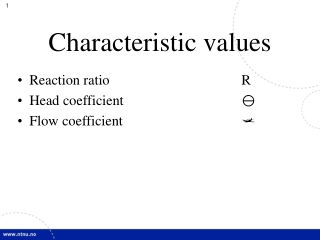DownloadDownload PresentationCharacteristic values

# Characteristic values

Télécharger la présentation## Characteristic values

- - - - - - - - - - - - - - - - - - - - - - - - - - - E N D - - - - - - - - - - - - - - - - - - - - - - - - - - -
##### Presentation Transcript

1. Characteristic values • Reaction ratio R • Head coefficient y • Flow coefficient j

2. Reaction ratio for hydraulic machines The reaction ratio can be defined as the pressure fraction of the total net specific energy which is converted to mechanical energy in the runner.

3. Reaction ratio for a Pelton turbine Example for a Pelton turbine : cu1 = 1,0 cu2 = 0 u1 = 0,5 cm1 = cm2 R = 0,0

4. Reaction ratio for a Francis turbine Example for a Francis turbine: cu1 = 0,72 cu2 = 0 u1 = 0,69 R = 0,52

5. Inlet Runner Outlet guide vanes Inlet guide vanes Outlet Draft tube Outlet runner Energy converted in the turbine Reference line

6. Reaction ratio for a Kaplan turbine Example for a Kaplan turbine: cu1 = 0,6 cu2 = 0 u1 = 0,83 R = 0,64

7. u1 w1 c1 w1 u1 c1 c2 w2 Aksialturbin R=0 w u2

8. w1 u1 c1 u2 c2 w2 Aksialturbin R=0,5 w

9. Reaction ratio The reaction ratio can be defined as the ratio between the enthalpy difference over the runner and the total change of enthalpy. p3 p3 h h p2 p2 3 0 Guidevanes Guidevanes p1 p1 1 Runner Runner 2 2 1 s s

10. Head coefficient for hydraulic machines

11. Flow coefficient for hydraulic machines

12. Source: Strømningsmaskiner, Bind 3, Jan M. Øverli